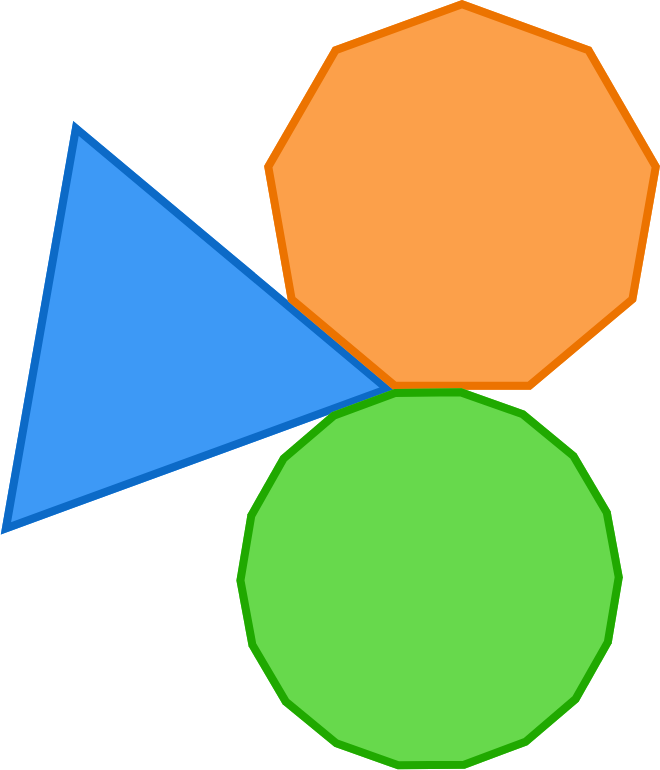# A Triangle and Two Regular Convex Polygons

Geometry Level 2

An equilateral triangle and 2 other regular polygons share a single vertex in such a way that the three shapes completely cover the $360^\circ$ of space surrounding the vertex without overlapping.

What is the largest possible number of sides that one of the regular polygons can have?An equilateral triangle, regular 9-gon, and regular 18-gon can cover all $360^\circ$ around a single vertex. Is 18 the maximum number of sides one of these regular polygons can have?

×